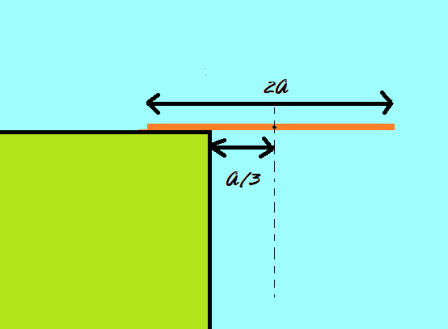# Save the rodA rod of mass $\displaystyle{M}$ and length $\displaystyle{2a}$ is placed horizontally on the edge of a table. Initially, the centre of mass of the rod is at a distance of $\displaystyle{^a/_3}$ from the edge. The rod is released from the rest. The rod slips after it has turned through an angle $\displaystyle{ \theta }$. The coefficient of friction $\displaystyle{\mu}$ between the rod and the table can be expressed as $\dfrac{a}{b} \tan \theta.$ Find $\displaystyle{a+b.}$

Note

• $a$ and $b$ are positive, co-prime integers.
• Liked it? Go here for more.
×

Problem Loading...

Note Loading...

Set Loading...